# Check ensemble of OpenMM temperature replica exchange simulations

Note: This notebook can be run locally by cloning the Github repository. The notebook is located in doc/examples/openmm_replica_exchange.ipynb. The input and output files of the simulation are located in doc/examples/simulation_results/openMMTemperatureReplicaExchange/. Be aware that probabilistic quantities such as error estimates based on bootstrapping will differ when repeating the analysis.

:

# enable plotting in notebook
%matplotlib notebook


Ensemble validation is particularly useful for validating enhanced sampling methods such as temperature replica exchange (parallel tempering) molecular dynamics simulations. In replica exchange MD, configurational swaps are attempted periodically between simulations running concurrently in separate simulation cells at different temperatures. Since the acceptance criteria for exchange moves depends on the energies of each replica involved, it is critical that the energy distributions are correct at all thermodynamic states used the parallel tempering scheme.

In this example, parallel tempering simulations on a simple coarse-grained oligomer system were run using the openmmtools framework. 6 temperature states spaced logarithmically over the range of 300K to 500K, over which the oligomer undergoes a transition from a helix to unfolded state.

:

import numpy as np
import physical_validation

import openmmtools.multistate
import simtk.unit

Warning: importing 'simtk.openmm' is deprecated.  Import 'openmm' instead.


For convenience, we will use native physical_validation units:

:

energy_unit = simtk.unit.kilojoule_per_mole
length_unit = simtk.unit.nanometer
volume_unit = length_unit ** 3
temperature_unit = simtk.unit.kelvin
pressure_unit = simtk.unit.bar
time_unit = simtk.unit.picosecond
kb = simtk.unit.MOLAR_GAS_CONSTANT_R.value_in_unit(energy_unit / temperature_unit)


Create the UnitData object which we will use to inform physical_validation of our choices. Note that the conversion factors will all be 1.0, since we’re using native units. The notation here is more general though, and would allow to change any unit set in the previous cell.

:

unit_data = physical_validation.data.UnitData(
kb=kb,
energy_conversion=energy_unit.conversion_factor_to(simtk.unit.kilojoule_per_mole),
length_conversion=length_unit.conversion_factor_to(simtk.unit.nanometer),
volume_conversion=volume_unit.conversion_factor_to(simtk.unit.nanometer ** 3),
temperature_conversion=temperature_unit.conversion_factor_to(simtk.unit.kelvin),
pressure_conversion=pressure_unit.conversion_factor_to(simtk.unit.bar),
time_conversion=time_unit.conversion_factor_to(simtk.unit.picosecond),
energy_str=energy_unit.get_symbol(),
length_str=length_unit.get_symbol(),
volume_str=volume_unit.get_symbol(),
temperature_str=temperature_unit.get_symbol(),
pressure_str=pressure_unit.get_symbol(),
time_str=time_unit.get_symbol(),
)


We first read the replica exchange output file and extract the thermodynamics states used in the simulation. We will use these states to create the EnsembleData objects which inform physical_validation of the sampled ensembles. Note that the example simulations were performed at constant temperature in a non-periodic box. We will therefore set the (undefined) volume of the NVT ensemble to -1.

:

output_data = "simulation_results/openMMTemperatureReplicaExchange/output/output.nc"
reporter = openmmtools.multistate.MultiStateReporter(output_data, open_mode="r")

num_states = len(states)
ensemble_data = [
physical_validation.data.EnsembleData(
ensemble="NVT",
natoms=state.n_particles,
volume=-1,
temperature=state.temperature.value_in_unit(temperature_unit),
)
for state in states
]

Warning: The openmmtools.multistate API is experimental and may change in future releases


We will then read the replica energies and the state indices. Note that

• replica_state_indices[replica, step] denotes the thermodynamics state index sampled by replica replica during step step

• replica_energies[replica, state, step] is the reduced potential of replica replica at state state during step step

:

analyzer = openmmtools.multistate.ReplicaExchangeAnalyzer(reporter)
replica_energies, _, _, replica_state_indices = analyzer.read_energies()

Warning: The openmmtools.multistate API is experimental and may change in future releases


For our analysis, we are only interested in the energies at the states the replicas were sampling at, rather than at all states. It will also make our remaining analysis easier if these energies are organized as time series per state rather than time series per replica.

Also, the replica energies are stored in reduced potential. For our analysis, we are interested in the non-reduced form, which we can obtain by multiplying the result by kT of the respective thermodynamic state.

:

# Prepare array of kT values
kT = np.array(
[kb * state.temperature.value_in_unit(temperature_unit) for state in states]
)

total_steps = replica_energies.shape
potential_energies = []

for state in range(num_states):
state_energy = np.zeros(total_steps)
for step in range(total_steps):
# Find the replica which sampled at state state during step step
state_energy[step] = replica_energies[
np.nonzero(replica_state_indices[:, step] == state), state, step
]
# Append non-reduced potential energy time series
potential_energies.append(state_energy * kT[state])


We now have all the required information to create a SimulationData object for each separate state:

:

simulation_data = []
for ensemble, potential_energy in zip(ensemble_data, potential_energies):
simulation_data.append(
physical_validation.data.SimulationData(
units=unit_data,
ensemble=ensemble,
observables=physical_validation.data.ObservableData(
potential_energy=potential_energy
),
)
)


We can now run the ensemble validation on all adjacent temperature pairs:

:

for simulation_lower, simulation_upper in zip(
simulation_data[:-1], simulation_data[1:]
):
physical_validation.ensemble.check(
data_sim_one=simulation_lower, data_sim_two=simulation_upper, screen=True
)

After equilibration, decorrelation and tail pruning, 4.20% (126 frames) of original Trajectory 1 remain.
After equilibration, decorrelation and tail pruning, 16.33% (490 frames) of original Trajectory 2 remain.
Overlap is 95.2% of trajectory 1 and 91.8% of trajectory 2.
Rule of thumb estimates that dT = 58.0 would be optimal (currently, dT = 32.3)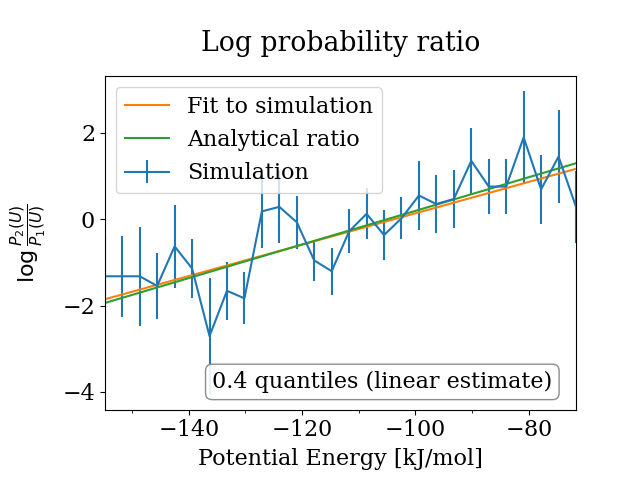==================================================
Maximum Likelihood Analysis (analytical error)
==================================================
Free energy
5.40765 +/- 0.60277
Estimated slope                  |  True slope
0.038921  +/- 0.005296       |  0.038936
(0.00 quantiles from true slope)
Estimated dT                     |  True dT
32.3   +/- 4.4               |  32.3
==================================================
After equilibration, decorrelation and tail pruning, 16.33% (490 frames) of original Trajectory 1 remain.
After equilibration, decorrelation and tail pruning, 15.93% (478 frames) of original Trajectory 2 remain.
Overlap is 95.7% of trajectory 1 and 92.5% of trajectory 2.
Rule of thumb estimates that dT = 63.9 would be optimal (currently, dT = 35.7)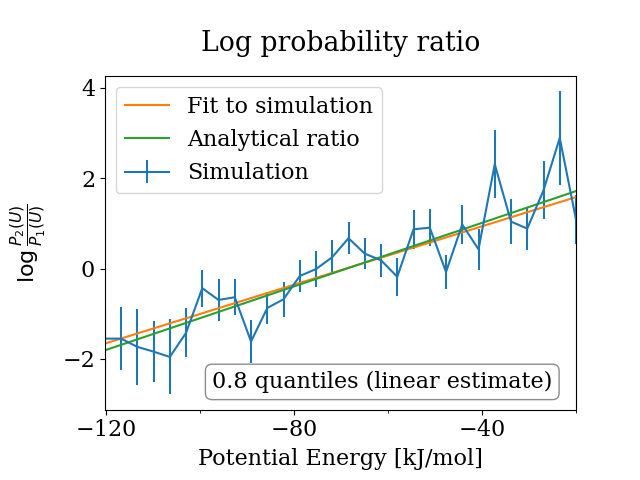==================================================
Maximum Likelihood Analysis (analytical error)
==================================================
Free energy
2.36236 +/- 0.21589
Estimated slope                  |  True slope
0.035230  +/- 0.002913       |  0.035155
(0.03 quantiles from true slope)
Estimated dT                     |  True dT
35.8   +/- 3.0               |  35.7
==================================================
After equilibration, decorrelation and tail pruning, 15.93% (478 frames) of original Trajectory 1 remain.
After equilibration, decorrelation and tail pruning, 9.20% (276 frames) of original Trajectory 2 remain.
Overlap is 96.2% of trajectory 1 and 93.1% of trajectory 2.
Rule of thumb estimates that dT = 71.6 would be optimal (currently, dT = 39.6)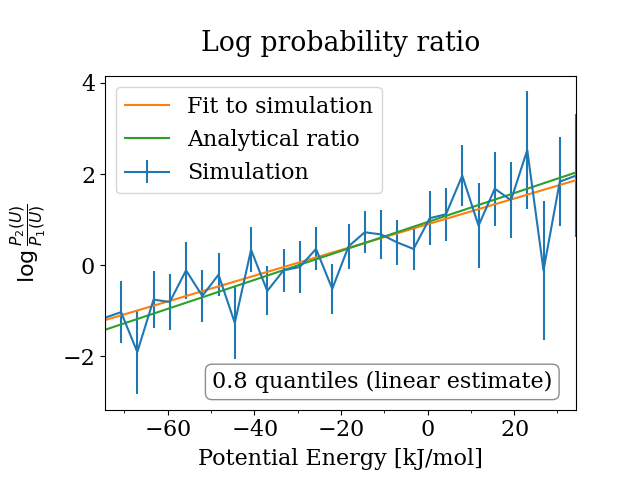==================================================
Maximum Likelihood Analysis (analytical error)
==================================================
Free energy
0.34585 +/- 0.14426
Estimated slope                  |  True slope
0.030943  +/- 0.003609       |  0.031740
(0.22 quantiles from true slope)
Estimated dT                     |  True dT
38.6   +/- 4.5               |  39.6
==================================================
After equilibration, decorrelation and tail pruning, 9.20% (276 frames) of original Trajectory 1 remain.
After equilibration, decorrelation and tail pruning, 6.30% (189 frames) of original Trajectory 2 remain.
Overlap is 81.2% of trajectory 1 and 97.4% of trajectory 2.
Rule of thumb estimates that dT = 81.0 would be optimal (currently, dT = 43.8)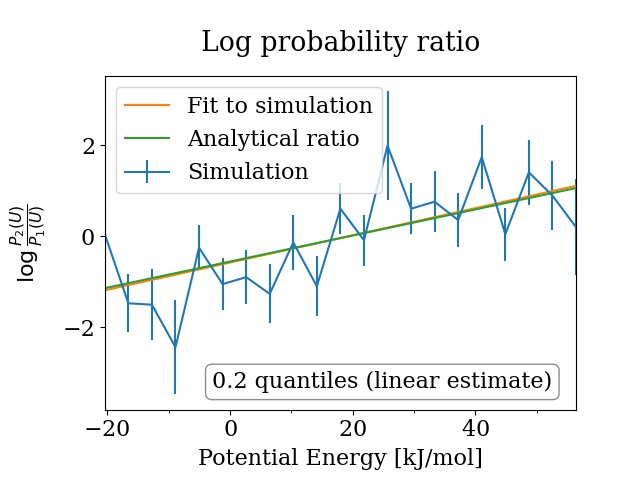==================================================
Maximum Likelihood Analysis (analytical error)
==================================================
Free energy
-0.79533 +/- 0.14068
Estimated slope                  |  True slope
0.030647  +/- 0.003797       |  0.028658
(0.52 quantiles from true slope)
Estimated dT                     |  True dT
46.9   +/- 5.8               |  43.8
==================================================
After equilibration, decorrelation and tail pruning, 6.30% (189 frames) of original Trajectory 1 remain.
After equilibration, decorrelation and tail pruning, 7.00% (210 frames) of original Trajectory 2 remain.
Overlap is 92.6% of trajectory 1 and 100.0% of trajectory 2.
Rule of thumb estimates that dT = 96.4 would be optimal (currently, dT = 48.6)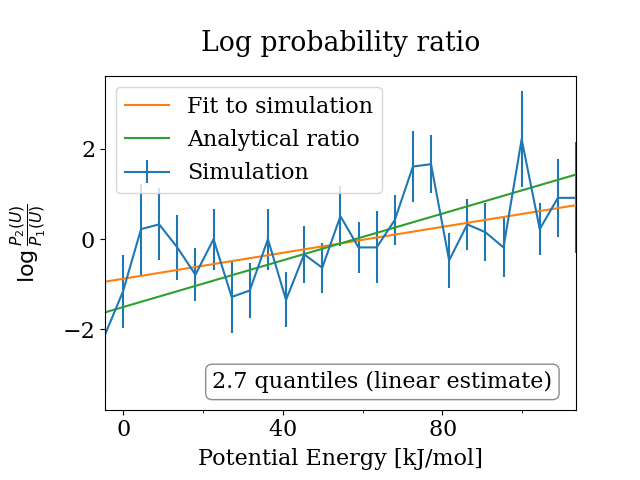==================================================
Maximum Likelihood Analysis (analytical error)
==================================================
Free energy
-0.96215 +/- 0.23485
Estimated slope                  |  True slope
0.019539  +/- 0.003492       |  0.025875
(1.81 quantiles from true slope)
Estimated dT                     |  True dT
36.7   +/- 6.6               |  48.6
==================================================


In this analysis, we observe satisfactory dependence of the ensemble distribution on the temperature. As a rule of thumb, if the true interval is not within about 2-3 standard deviations of the estimated interval, the trajectory is unlikely to have been sampled from the expected ensemble. All intervals are below 2 quantiles from the true slope in the maximum likelihood analysis, the first four are around or below 0.5 quantiles from the true slope.